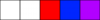# Tile Base Collision Line

#### curato

##### Member
GM Version: 2.3.1.409
Target Platform: ALL

Summary:
This will show how to make a tile base collision line that will detect if a specific tile is present long the line.

Tutorial:
I made a little sample project (above) that when the player tries to fire it determines if the path is clear of a specific tile and if so, will shoot a bullet otherwise you just get a click noise. This could be used among other things to keep you AI from seeing through tile walls or trying to shoot when you clearly can’t be shot. However, we are going to keep it simple.
This is the tileset I am using for the tutorial. I show it to say a lot of tutorials just use !=0 and that cheats you out of options different positions can be used for anything. They could block the player but not bullets like a pit for example, but that is beyond the scope the tutorial. The important part is we are going to look for one specific position in this case 1. (I used 2 for the floor in the sample and you can see that it is ignored entirely.Now the player moves forward and back with up and down arrows and rotates with the left right, but how can we check for the collision at any angle. we are going to use a function we called tileCollisionLine.
Here is the how we call it.
tileCollisionLine(xpos,ypos,size,tileset,tilepos,Length,Dir) // return true/false
• xpos is the x position of the start of the line.
• ypos is the y position of the start of the line.
• size is the amount of distance to move to check along the line for the next check (make this less than the tile size.)
• tileset is the tileset we are searching. Make sure to declare one with layer_tilemap_get_id
• tilepos the position in the tileset you are looking for (in this case 1 could be any one of the tiles)
• Length the distance away from the point to check
• Dir the angle we are facing in degrees
Now for the function:
GML:
``````/// @description tileCollisionLine(xpos,ypos,size,tileset,tilepos,Length,Dir)
function tileCollisionLine(argument0,argument1,argument2,argument3,argument4,argument5,argument6)
{
var xpos = argument0; // start position x coordinate
var ypos = argument1; // start position y coordinate
var size = argument2; // size of check interval (less than tile size)
var tileset = argument3; // which tileset is being checked.
var tilepos = argument4; // which tile position in tileset is being checked for
var Length = argument5; // length of line from xpos and ypos to look for a position
var Dir = degtorad(argument6); // current direction converted to radians for use

var sinDir = sin(Dir); // precalc outside the loop as it doesn't change
var cosDir = cos(Dir); // precalc outside the loop as it doesn't change

for (var i = 0; i<= Length; i += size)  // check the length of the line in intervals determined by size
{
var xi = xpos + i * cosDir; // x position along line at i distance
var yi = ypos - i * sinDir; // y position along line at i distance

if (tilemap_get_at_pixel(tileset, xi, yi) == tilepos) then // if tile position is found then
{
return true; // found a collision
exit; // no need to run this anymore so get out
}
}
return false; // didn't find a collision along the line so return false
}``````
So this function first breaks out the arguments as mentioned above. Of special interest is the Dir I convert to radian for use for some good old trigonometric functions.
GML:
``````    var sinDir = sin(Dir); // precalc outside the loop as it doesn't change
var cosDir = cos(Dir); // precalc outside the loop as it doesn't change``````
I added this to reduce calculation as they do not change during the loop

Code:
``for (var i = 0; i<= Length; i += size)``
We are going to loop through increasing the search by size until we reach the full search length

Code:
``````var xi = xpos + i * cosDir; // x position along line at i distance
var yi = ypos - i * sinDir; // y position along line at i distance``````
We are going to calculate the position of the line on a circle of the search size based on the direction we are facing.

Code:
``````if (tilemap_get_at_pixel(tileset, xi, yi) == tilepos) then // if tile position is found then
{
return true; // found a collision
exit; // no need to run this anymore so get out
}``````
We check the position along the line and if it returns our requested tilepos then we found it. we are going to return true and we are going to exit because we found what we are looking for.

Code:
``return false; // didn't find a collision along the line so return false``
This is the fall through if we do our search and don't find anything then we are just going to return false because we didn’t find anything.

Last edited:
••Chourando, RefresherTowel and EvanSki

#### EvanSki

##### Raccoon Jam Host
@rytan451 Helped me out a while ago with this and wrote a decent script for doing so as well

Thanks to you both for the info and tutorial

•curato

#### curato

##### Member
I thought that was you that asked for something like that. I thought I remembered reading that some where. I was messing with improving my tile functions and got carried away and wrote this and was like I remember someone asking for this.

•EvanSki